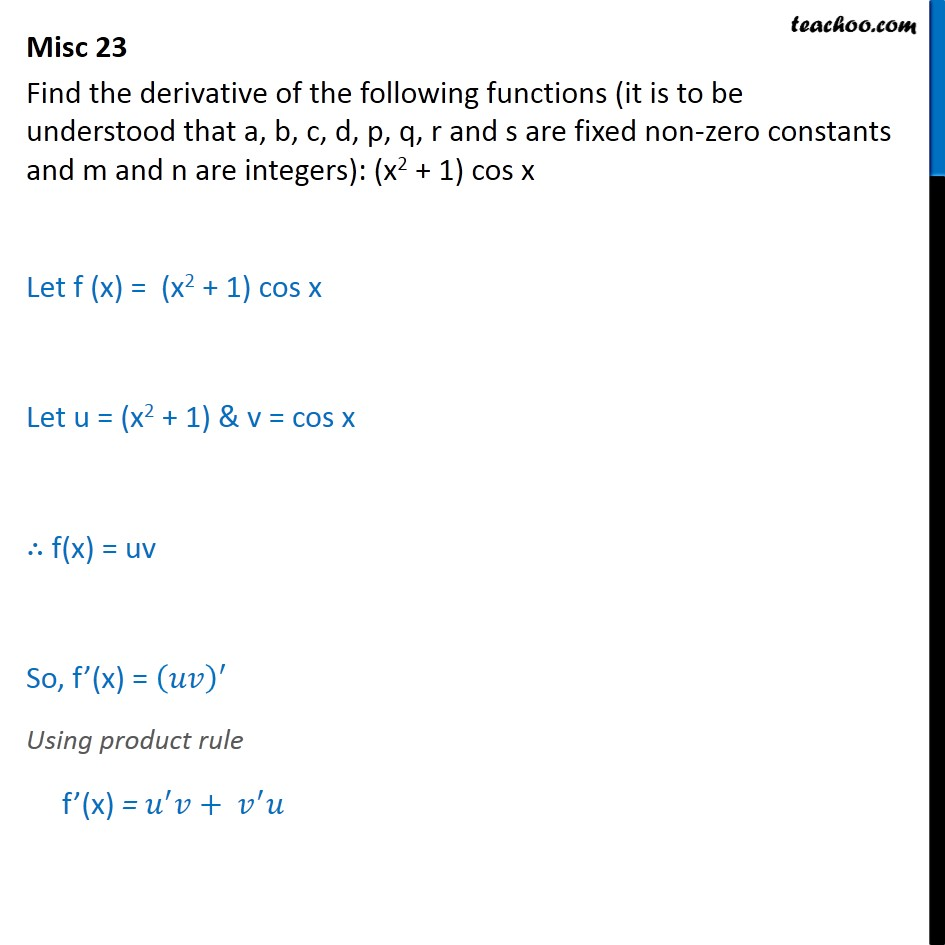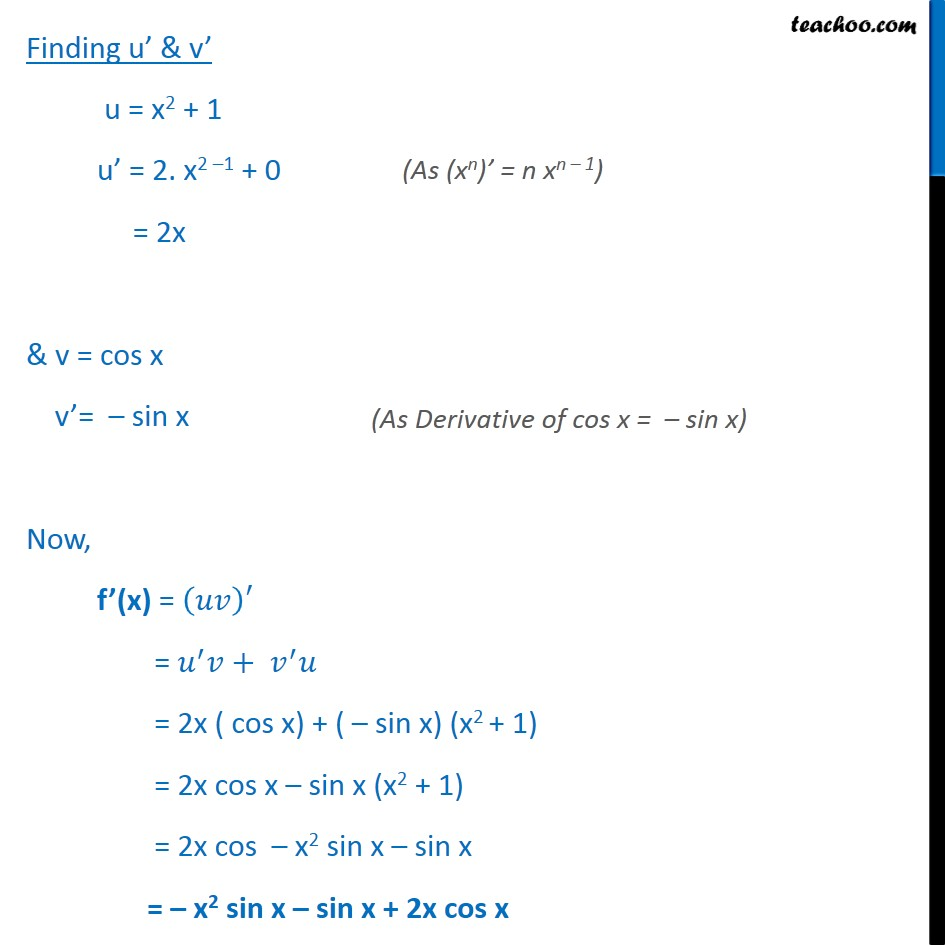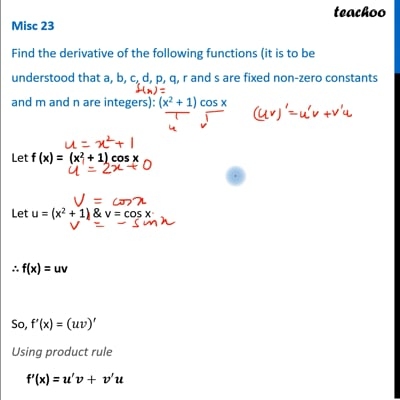Miscellaneous

Chapter 13 Class 11 Limits and Derivatives
Serial order wiseThis video is only available for Teachoo black users

Get live Maths 1-on-1 Classs - Class 6 to 12

### Transcript

Misc 23 Find the derivative of the following functions (it is to be understood that a, b, c, d, p, q, r and s are fixed non-zero constants and m and n are integers): (x2 + 1) cos x Let f (x) = (x2 + 1) cos x Let u = (x2 + 1) & v = cos x f(x) = uv So, f (x) = Using product rule f (x) = + Finding u & v u = x2 + 1 u = 2. x2 1 + 0 = 2x & v = cos x v = sin x Now, f (x) = = + = 2x ( cos x) + ( sin x) (x2 + 1) = 2x cos x sin x (x2 + 1) = 2x cos x2 sin x sin x = x2 sin x sin x + 2x cos x